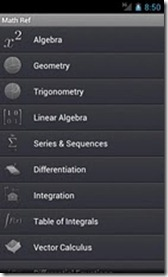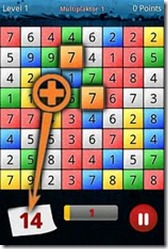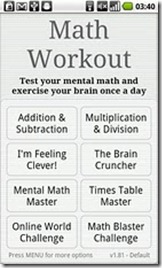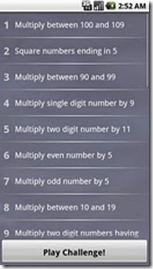Editor Ratings:
User Ratings:
[Total: 0 Average: 0]

Here are 5 free Android math apps which will help you to exercise and improve your mathematical skills. Lets get into details of each math Android app.

## Math Ref FreeAs the name implies Math Ref Free is one of the most popular Android math apps which works as a mathematical reference app. This Android math app covers a lot of mathematical topics and equations including Trigonometry, Geometry, Integration, Derivatives, Algebra, Statistics and Probability.

Math Ref Free also includes many formula related to Physics. This Android math app is free version of Math Ref app costing \$0.99 and sized 19 MB, while Math Ref Free is just 8.3 MB download. The double sized package includes added Chemistry, Physics and Mathematical formulae and equations like Vector Calculus, Algorithms, Discrete Mathematics, etc. Math Ref Free is a must have math app for high-school and college students.

iPhone users can check out iPhone Math Apps reviewed by us.

## Math ManiacMath Maniac is a puzzle math app. This math game app has a board containing numbers from 1 to 9. Our task in the game is to tap on the numbers to add them and so that the final sum is the number displayed at the lower left corner of the screen. To do this just 10 seconds are given and 10 seconds are enough to do so. The count-down timer is also displayed at the lower end of the screen. The difficulty level in this math app for Android increases slowly, along with increasing the player’s mathematical skills.

Check out other Android Apps reviewed by us.

## Math WorkoutMath Workout has got as popular as Gym Workout apps on Android Market with more than 3 million downloads. This free math app for Android is designed to exercise the brain for a few minutes daily.

Math Workout includes many sub-exercises and games. The gameplay is challenging. The user has to react fast in order to delay the Game Over message. Some exercises in Math Workout are Time Table Master, Math Blaster Challenge, The Brain Cruncher and Mental Math Master. Math Workout is an ad-supported app. The ad-free version is priced around \$2.

## Kids Numbers and Math FreeKids Numbers and Math Free is an Android math app for kids. With a child-friendly interactive interface this Android math app makes them to learn the initial math skills like counting, addition and subtraction. We can set the minimum and maximum number. However, with the premium Kids and Math app costing \$2.99, the number range is lifted up to 20.

## Fun Math Tricks LiteWant to learn some cool Math tricks? Get the Fun Math Tricks Lite app for Android. This Android math app is designed for the users to learn some advanced tricks so that they can save time while in professional entrance examination like MCAT and GMAT. The tricks taught in this app are like getting the square root of a number(say 58) with a short and quick method, instead of wasting time in multiplication. Sounds cool!

 Editor Ratings: User Ratings:[Total: 0 Average: 0] Works With: Android Free/Paid: Free